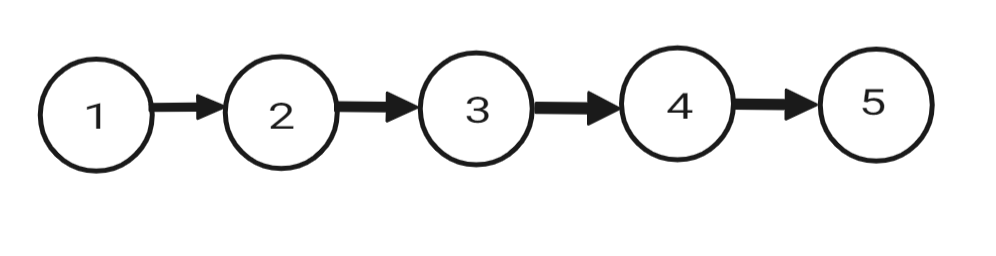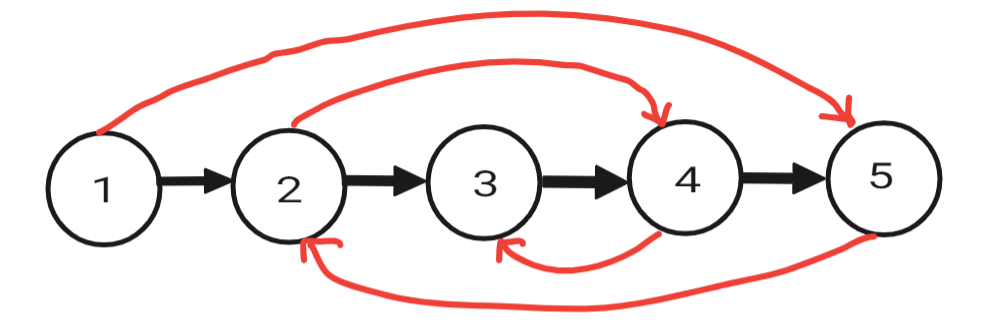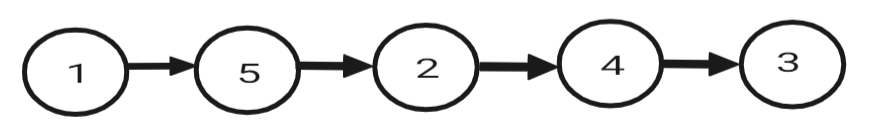# Reorder List

Problem Statement: You are given the head of a singly linked list.

```The list can be represented as :
L0 → L1 → … → Ln - 1 → Ln
Reorder the list to be on the following form:
L0 → Ln → L1 → Ln - 1 → L2 → Ln - 2 → …
```

Examples:

Example 1:

Input: N = 5, List = {1, 2, 3, 4, 5}Output:Example 2:

Input: N = 4, List = {1, 2, 3, 4}

Output:

### Solution

Disclaimer: Don’t jump directly to the solution, try it out yourself first.

Approach: The solution to this problem can be divided into three steps:

• Find the midpoint and split the linked list into two halves from there.
• Now, Reverse the second half of the list.
• Now, connect both lists (A and B) such that  A0 -> B0 -> A1 -> B1 -> A2 -> B2 ….

Let’s dry run the above approach on example 1.

Step – 1: Split the Linked List from the Midpoint

Step – 2: Reverse the Second List

(Reversed List)

Step – 3 : Connect both lists (A and B) such that  A0 -> B0 -> A1 -> B1 -> A2 -> B2 ….

Output :Code:

## C++ Code

``````class Solution {
public:

{

while(fast->next and fast->next->next){
slow = slow->next;
fast = fast->next->next;
}

return slow;
}

ListNode*prev = NULL;
ListNode*forw = NULL;

while(curr){
forw = curr->next;
curr->next = prev;
prev = curr;
curr = forw;
}

return prev;
}

void reorderList(ListNode* head) {

return;
}

ListNode*k = reverse(mid->next);
mid->next = NULL;

ListNode*c2 = k;
ListNode*f1 = NULL;
ListNode*f2 = NULL;

while(c1!=NULL and c2!=NULL)
{
f1 = c1->next;
f2 = c2->next;

c1->next = c2;
c2->next = f1;

c1 = f1;
c2 = f2;
}
}
};
``````

Time Complexity : O(N) [Middle of List] + O(N/2) [Reversing Second Half] + O(N/2) [Connecting both lists]  = O(2N)  = O(N)

Space Complexity : O(1)

## Java Code

``````class Solution {

ListNode slow = head;
ListNode fast = head;

while(fast.next != null && fast.next.next != null){
slow = slow.next;
fast = fast.next.next;
}
return slow;
}

ListNode curr = head;
ListNode prev = null;
ListNode forw = null;

while(curr != null){
forw = curr.next;
curr.next = prev;
prev = curr;
curr = forw;
}
return prev;
}

public void reorderList(ListNode head) {
if(head == null || head.next == null)
return;

ListNode mid = middle(head);
ListNode k = reverse(mid.next);
mid.next = null;

ListNode c1 = head;
ListNode c2 = k;
ListNode f1 = null;
ListNode f2 = null;

while(c1 != null && c2 != null){
f1 = c1.next;
f2 = c2.next;

c1.next = c2;
c2.next = f1;

c1 = f1;
c2 = f2;
}
}
}
``````

Time Complexity : O(N) [Middle of List] + O(N/2) [Reversing Second Half] + O(N/2) [Connecting both lists]  = O(2N)  = O(N)

Space Complexity : O(1)

Special thanks to Sagar Srivastava for contributing to this article on takeUforward. If you also wish to share your knowledge with the takeUforward fam, please check out this article